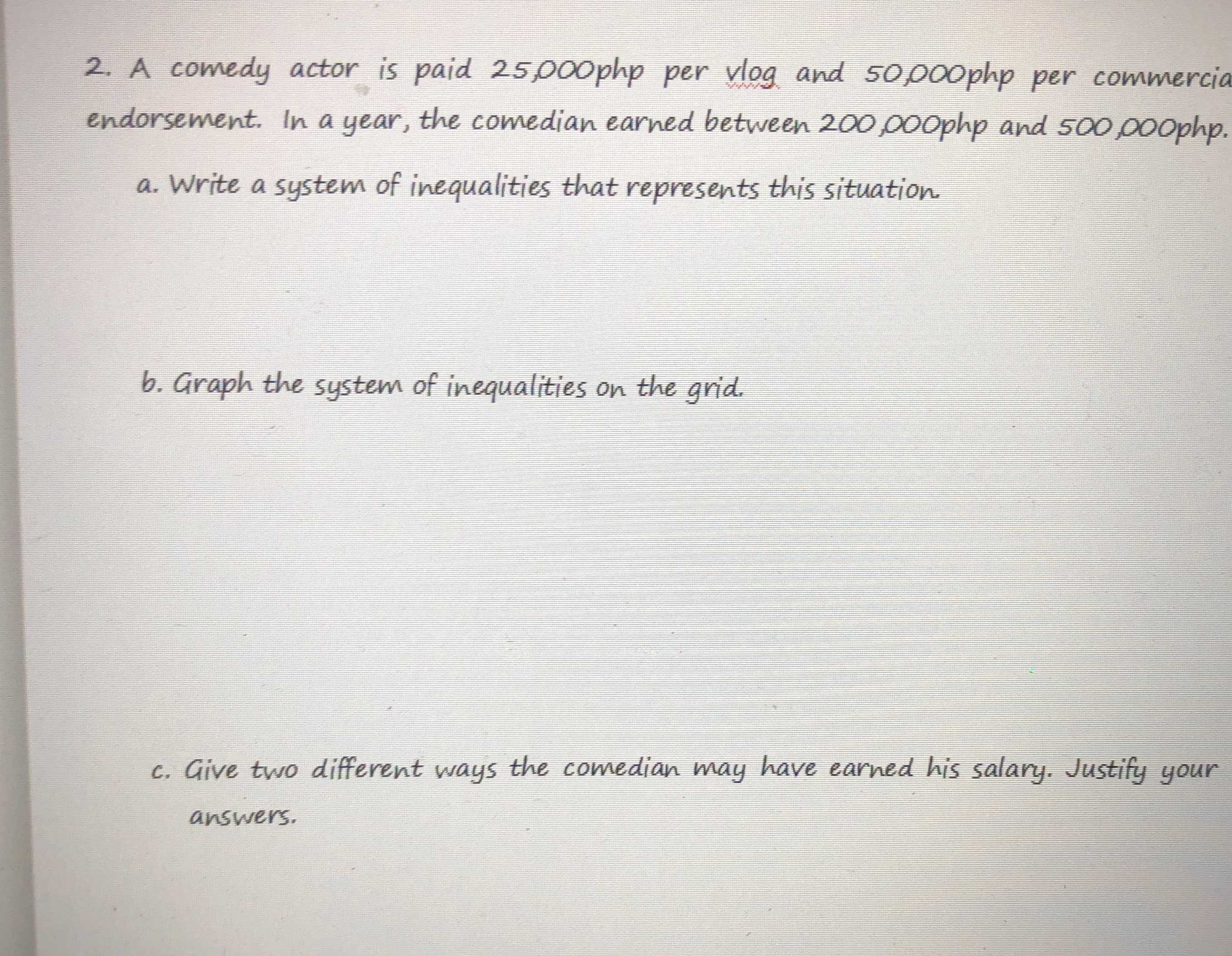### ¿Todavía tienes preguntas de matemáticas?

Pregunte a nuestros tutores expertos
Algebra
Pregunta2. A comedy actor is paid $$25,000 p h p$$ per vlog and $$50,000 p h p$$ per commercia endorsement. In a year, the comedian earned between $$200,000 p h p$$ and $$500,000 p h p$$ .

a. Write a system of inequalities that represents this situation

b. Graph the system of inequalities on the grid.

c. Give two different ways the comedian may have earned his salary. Justify your answers.

200000 $$\le$$25000x $$\le$$ 500000
200000 $$\le$$ 50000y $$\le$$ 500000